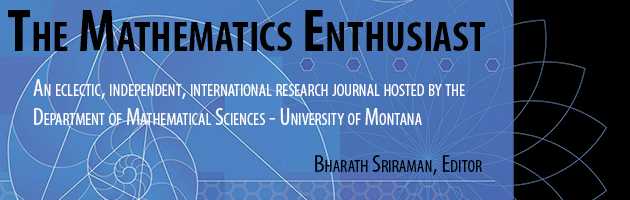•
•2

1

#### Abstract

mathematics curriculum. As seen in her paper, the solution of the cubic essentially involves the use of a clever substitution in the general cubic in order to reduce it into a cubic without the square term, which in turn is factorable as a quadratic provided one makes another substitution. A natural question to ask ourselves, which mathematicians in the colorful theory of equations undoubtedly did as well, is how far can we push this technique of cle ver substitutions to solve higher degree equations. These substitutions are called Tschirnhaus transformations and have a pattern of the form x = y -a/n. In Ballou’s (2005) paper the transformation used in the cubic was x = y - a/3 which allowed us to get rid of the so-called pesky x2 term. For the general quartic the transformation is x = y –a/4 which in turn transforms the equation into a cubic solveable by Cardano’s method. The question is what happens when we try our technique of Tschirnhaus transformations into the general quintic. To find out we first need to make a sidetrack into a little history packed with drama.

72

76

COinS